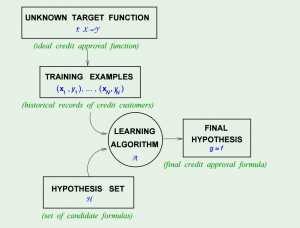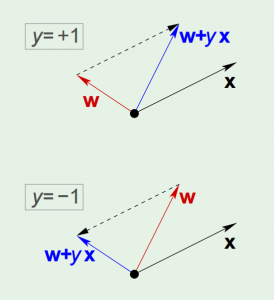## Learning from Data 2: Is Learning Feasible?

Learning from Data is a Machine Learning MOOC taught by Caltech Professor Yaser Abu-Mostafa. I took the course in 2014 through edX. It was a great course, well-organized and into the details.

Recently I have been reviewing the notes and the summary of the course, and I thought I might just post them here. All my code used for the homework can be found at https://github.com/Chaoping/Learning-From-Data.

Lecture 2: Is Learning Feasible?

Lecture 2 starts with a question of probability: Can a sample tell anything about the population? In probability, the answer is yes – and we know the bigger the sample, the better it represents the population. Now instead of confidence interval, here we use another way to measure the sample size – precision trade off, that is, the Hoeffding’s inequality: $$\mathbb{P}[|\mu – \nu |>\epsilon] \le 2e^{-2\epsilon^2N}$$

With Hoeffding’s Inequality, we can say that “$\mu = \nu$” is “P.A.C” true (probably, approximately, correct). “Probably” because the probability of violation is small, and “approximately” because $\epsilon$ is small (with good size of $N$).

Now, consider a learning problem, the only assumption is that, the data we have, is simply a sample, generated with a probability distribution. The analogy is that, we know how a hypothesis performs on the training set, and with Hoeffding’s Inequality we believe it should reflect how the hypothesis will work on the data we haven’t seen. By changing the notation of $\mu$ and $\nu$, we have $$\mathbb{P}[|E_\mathrm{in}(h) – E_\mathrm{out}(h) |>\epsilon] \le 2e^{-2\epsilon^2N}$$

However there is yet another problem – Hoeffding’s Inequality applies to each one of the hypothesis, but when we combine many hypotheses all together, it fails. It is because we “tried too hard”.

To solve it, we can give it a union bound. Since the final hypothesis $g$ is one element in the hypothesis set $H$, without giving any extra assumptions, we can safely say that $$\mathbb{P}[|E_\mathrm{in}(g) – E_\mathrm{out}(g) |>\epsilon] \le 2Me^{-2\epsilon^2N}$$

Now with $M$ being the number of hypotheses in $H$, we once again give it a bound.

## Learning from Data 1: The Learning Problem

Learning from Data is a Machine Learning MOOC taught by Caltech Professor Yaser Abu-Mostafa. I took the course in 2014 through edX. It was a great course, well-organized and into the details.

Recently I have been reviewing the notes and the summary of the course, and I thought I might just post them here. All my code used for the homework can be found at https://github.com/Chaoping/Learning-From-Data.

Lecture 1: The Learning Problem

Lecture 1 is the brief introduction of the course. It answered the question of “what is learning”. So the essence of learning is that:

• A pattern exists, which we are trying to find
• We cannot pin it down mathematically (otherwise we would not need to “learn”)
• We have data

And a learning problem can be formalized as:

• Input: $\mathbf{x}$
• Output: $y$
• Target function: $f: X \rightarrow Y$, which we do not know
• Data: $(\mathbf{x}_1, y_1), (\mathbf{x}_2,y_2),…,(\mathbf{x}_N,y_N)$ $$\downarrow$$
• Hypothesis: $g: X \rightarrow Y$, to approximate the target function.

In fact, to come up with the final hypothesis $g$, we are using a hypothesis set $H$, in which there are infinite hypotheses. A chart in the course slides shows the concept:The Hypothesis Set $H$ and the Learning Algorithm $A$ together are referred as the “learning model”.

Then the simplest example of learning is the “perceptron”, its linear formula $h \in H$ can be written as: $$h(x) = \mathrm{sign} ((\sum_{i=1}^{d}{w_i}{x_i})-t)$$ where t represents the threshold.

If we change the notation of $t$ to $w_0$, and introduce an artificial coordinate $x_0 = 1$, all of a sudden it can now be written as: $$h(x) = \mathrm{sign}(\sum_{i=0}^{d}{w_i}{x_i})$$ or equivalently, $$h(x) = \mathrm{sign}(\mathbf{w}^\intercal\mathbf{x})$$

The concept of perceptron’s learning algorithm is rather intuitive, that it tries to correct a random misclassified point every iteration, by: $$\mathbf{w} = \mathbf{w} + {y_n}\mathbf{x_n}$$ The correction can be visualized as the following:The algorithm keeps iterating, and eventually it will come to a point where all points are correctly classified, so long as the data is linearly-separable.

There are 3 types of learning:

• Supervised Learning: input, output
• Unsupervised Learning: input, no output
• Reinforcement Learning: input, grade for output

## Optimizing connectivity for users in China with reverse proxy

The game I am currently working on is a mobile strategy game. We have a server cluster located in the US to serve players around the world. It should suffice the needs – players in Europe and Oceania will experience a latency around 50 to 200 ms, but there is rarely any packet loss, so it is totally playable. Unfortunately it is a whole different story for players in China.

And really it has nothing to do with the censorship. Basically, China has the most internet users, but its global bandwidth is so limited. At peak time, many users can’t even visit foreign sites, let alone playing games on foreign servers. Dedicated players find their own solutions by subscribing to VPN-like accelerators, or using the value-added services called NOS (previously known as Global Delicate Network, 国际精品网, offered by the largest ISP, China Telecom). Those solutions utilize reserved global bandwidth, for example the CN2 backbone, which is primarily used by transnational corporations. The thing is, you can not expect every potential user of yours to have such service. So what about that we find a server that uses such reserved global bandwidth, and make it a reversed proxy for our servers in the US?

Preparation and Client

The first thing is to enable alternative connections in client. We want to enable players in China to choose to connect to the reverse proxy while keeping users in the rest of the world unchanged. Our approach is that when a user opens the game, it retrieves all possible connections, including the server cluster itself and reverse proxies – we call them nodes. On the client side, it determines whether the player is in China, if so, it reveals the node options to the user. All data will then be sent to and receive from the chosen node.

Reverse Proxy Solutions

There are many reverse proxy solutions. I have listed a few:

 HTTP TCP UDP iptables ✓ ✓ ✓ NGINX ✓ ✓ ✗ Apache ✓ ✗ ✗ HAProxy ✓ ✓ ✗

I will use HAProxy on Ubuntu 14.04 as an example:

Install HAProxy:

apt-get update
apt-get install haproxy


Configure the reverse proxy. Add the following to the config file /etc/haproxy/haproxy.cfg:

listen gameproxy 0.0.0.0:1337
mode tcp
option tcplog
server gameserver 123.123.123.123:1337


Start HAProxy using the config:

haproxy -f /etc/haproxy/haproxy.cfg


Done. Now this node works as if it were the server itself.

Also, instead of implement a reverse proxy yourself, there are similar services you can purchase, such as VnetLink. It provides fast and cheap services. However its uptime isn’t as good, and its pricing model gives me big headache.

Package RMySQL turns R to a powerful MySQL client. Below are the simple steps to use it:

install.packages("RMySQL")
library(RMySQL)


Connect to a MySQL database:

connection = dbConnect(MySQL(), host = "localhost", username = "root", password ="password", dbname = "databasename")


If you do not know the database name, you can omit it for now and use the following to find out all databases assigned to this user:

dbGetQuery(connection, "show databases")


To send queries:

# This sends the query but the result stays in MySQL server.
query = dbSendQuery(con, "query")

# Retrieve n rows of the result, and put it in a data.frame. If n is set to -1, it will retrieve all rows.
data = dbFetch(query, n)

# Clear the query on MySQL server side.
dbClearResult(query)


Another function allows you to do all the three steps – query, fetch and clear at once:

data = dbGetQuery(con, "query")


A good practice is always remembering to disconnect:

dbDisconnect(connection)